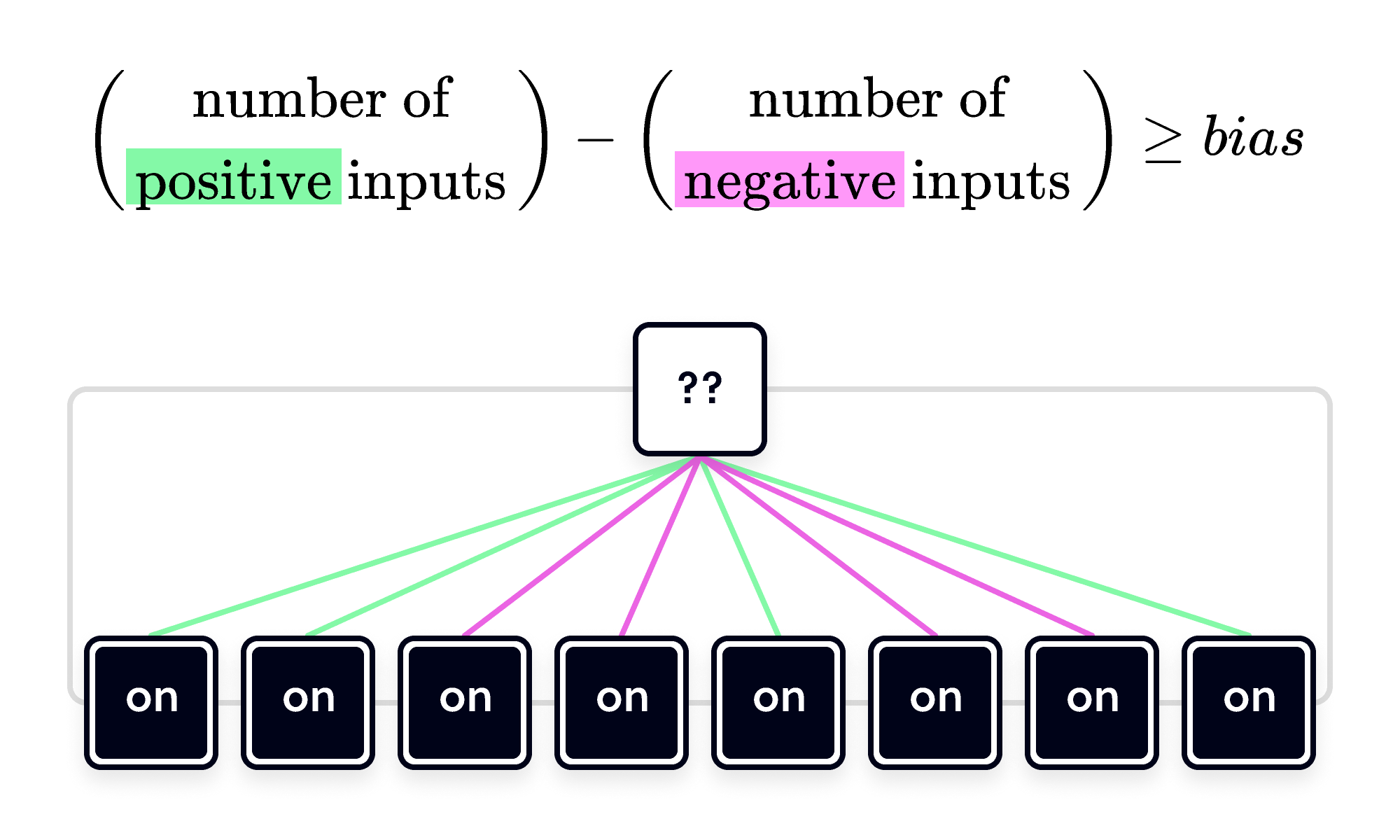Back

## Neuron Logic

The heart of a computer is Boolean logic. All of a computer's complexity ultimately rests on logical operations like $\mathbf{AND}, \mathbf{OR}, \mathbf{NOT},$ and $\mathbf{NAND}$ acting mechanistically on the information contained in bits.

Problems that break into a sequence of logical steps are ripe for solving with a computer. But what if the logic of a particular problem is beyond your reach?

Enter the artificial neural network.

An artificial neural network (ANN) is a system that can learn the logic of complex patterns in data. Its basic unit is the artificial neuron, inspired by simplified mathematical models of the neurons in animal brains.

Just as biological neurons send and receive electrical impulses to other neurons and change their own state based on the pulses they receive, the artificial neuron can be active $(\blacksquare)$ or inactive $(\square)$ based on the information it receives from its inputs.

The single neuron below has $3$ inputs, which can be on oroff. Clicking on an input will change its state.

The inputs with green edges drive the neuron toward its active state $(\blacksquare).$ The pink input has the opposite effect, inhibiting the neuron when it's on.

The neuron's output state is determined by summing the inputs: each green edge contributes $+1$ and pink one contributes $-1.$ (Only the inputs that are on are counted; inputs switched off have no effect.)

When the sum of the inputs equals or exceeds a particular value, called its bias, the neuron flips from $\square$ to $\blacksquare.$ The higher the bias, the greater the input needed to flip the neuron.

The leftmost input alone isn't enough to flip the neuron to $\blacksquare,$ but if you set both green inputs to on, their combined effect is. Likewise, the pink neuron lowers the total input, and it flips the output neuron back to its inactive state $(\square).$With this rule, a neuron can be configured to perform basic logical operations (or "gates") on the inputs. For example, the neuron below mimics the logical $\mathbf{AND}$ gate.

The neuron flips to $\blacksquare$ only if $\text{Input 1 } \mathbf{AND} \text{ Input 2}$ are on.Outputs of $\mathbf{AND}$ and $\mathbf{OR}$ gates for all four combinations of on and off.

By adjusting the bias, this neuron can replicate a completely different gate. For example, the $\mathbf{OR}$ gate flips to $\blacksquare$ if $\text{Input 1 } \mathbf{OR} \text{ Input 2}$ is on.

Because the $\mathbf{OR}$ gate is activated by a single positive input, its bias is lower than a configuration that mimics $\mathbf{AND}.$

On the neuron below, the bias is represented by the triangle on the meter showing different bias settings. You can set it higher or lower with the + and - buttons (click the meter to access). If you increase the bias to its highest setting, you should recover the $\mathbf{AND}$ gate.

Other logic gates are possible with negative inputs and negative bias settings. A neuron with a bias of zero or less is $\blacksquare$ even when none of the inputs are on. $($The center of the meter on the neuron corresponds to a bias of $0.)$

This doesn't mean a bias value that is zero or negative is useless. In fact, the $\mathbf{NOT}$ gate isn't possible with strictly positive bias values. This gate outputs the opposite of its single input:

# Today's Challenge

A $\mathbf{NAND}$ gate takes two inputs: if both inputs are on, it outputs $\square,$ otherwise it outputs $\blacksquare.$The neuron below has $4$ inputs: two positive (green) and two negative (pink). We can make different logic gates by using different pairs of the inputs; we can make $\mathbf{AND}$ and $\mathbf{OR}$ with the two green inputs, for example.

Which two inputs can you use to make a $\mathbf{NAND}$ gate?

Fun fact: Any Boolean logic gate can be constructed from a series of $\mathbf{NAND}$ gates, so by building a $\mathbf{NAND}$ gate using a neuron, you are showing that artificial neurons can carry out any logical function.Select one or more

×